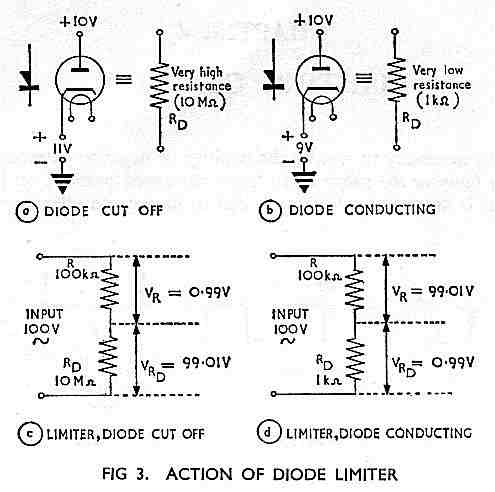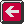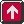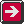Main Radar Home Radar theory Home AP3302 Pt3 Contents AP3302 Pt3 Section 2Contents AP 3302 Pt. 3 Section 2 CHAPTER 4 Limiting CircuitsTherefore when the input polarity is such that the diode is cut off the voltage developed across RD is much larger than that developed across R. For the figures given, the peak voltage across the diode is:- RD / RD + R x V = 10MW / 10MW + 100kW x 100 = 99.01V The peak voltage across the resistor is then 0. 99V (see Fig 3c). When the input polarity is such that the diode is conducting, the voltage developed across R is much larger than that developed across RD For the figures given, the peak voltage across R is:- R / R + RD x V = 100kW / 100kW + 1kW x 100 = 99.01V The peak voltage across the diode is then 0.99V (see Fig 3d). Thus practically the whole of the applied voltage is developed across either the resistor or the diode depending upon whether the valve is conducting or cut off. Series Limiters In a series limiter the diode is connected in series with the circuit to which we apply the output waveform, and the output voltage is taken across the resistor. Fig 4 shows the two forms of series limiter. If the input to the series negative limiter shown in Fig 4a is a sine wave which varies about earth the diode conducts only during the positive-going half-cycles and is cut off during the negative-going half-cycles. When the diode is conducting the voltage developed across it is negligible and the output voltage VR almost equals the input voltage Vin. When the diode is cut off VR is practically zero. This circuit therefore clips the portion of the input waveform which goes negative with respect to earth. If we turn the diode round we have a series positive limiter as shown in Fig 4b. In this circuit the diode can conduct only during the negative-going half-cycles and so the positive-going portion of the input waveform is clipped.Previous page To top of this page Next Page

Constructed by Dick Barrett
Email: